Miscellaneous

Chapter 10 Class 11 Straight Lines
Serial order wise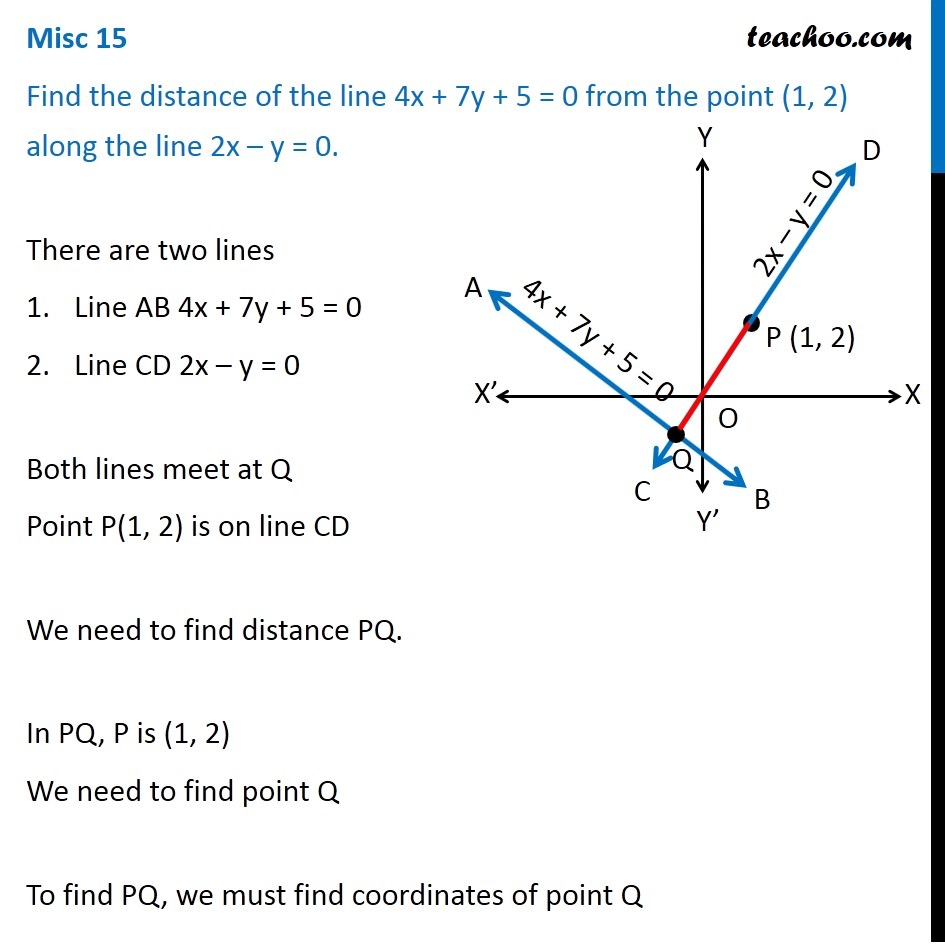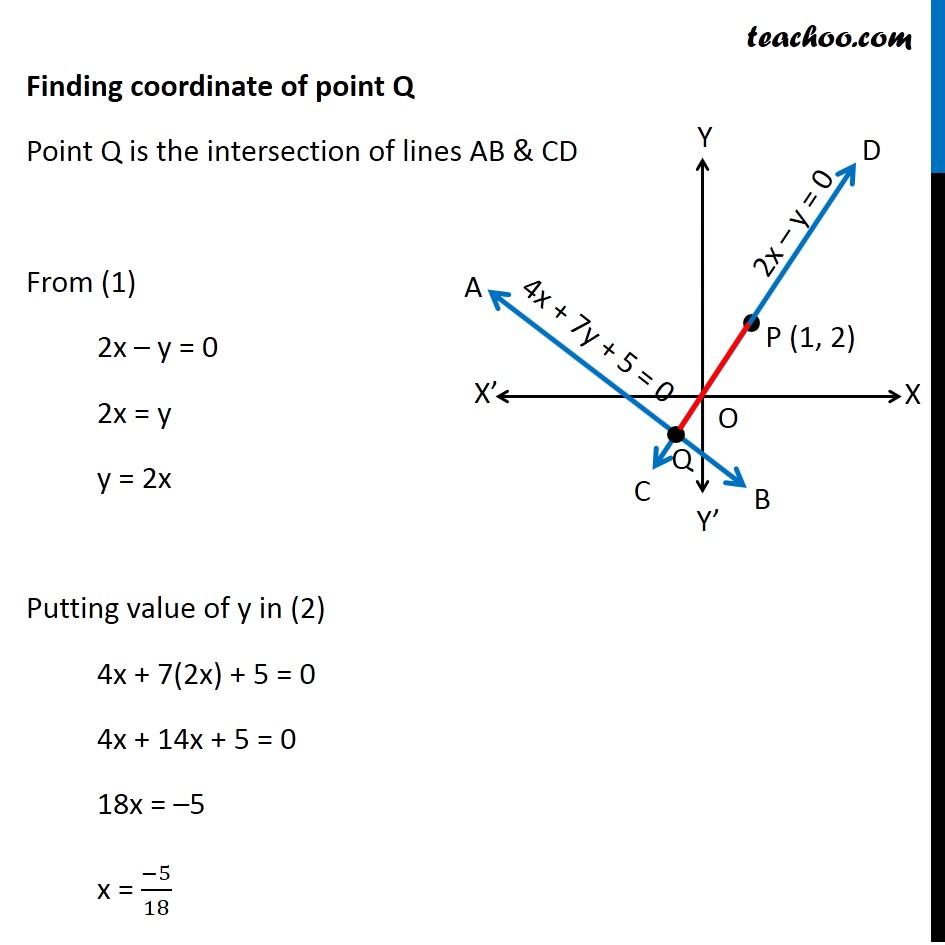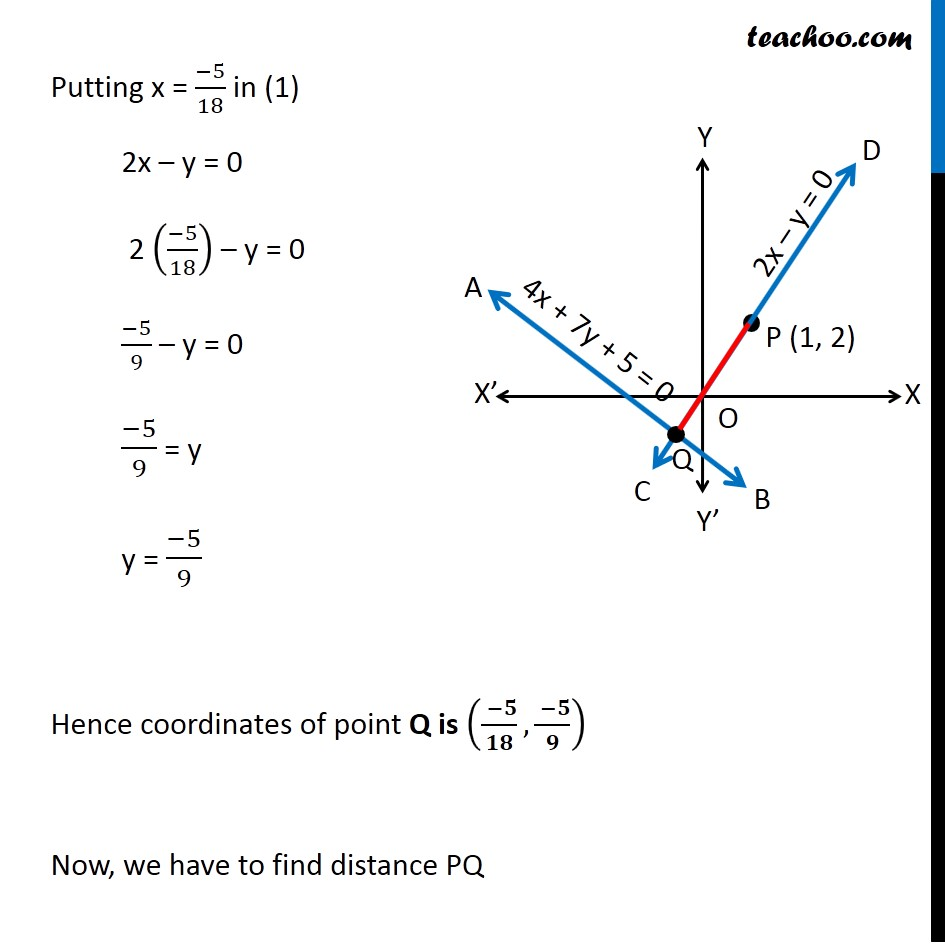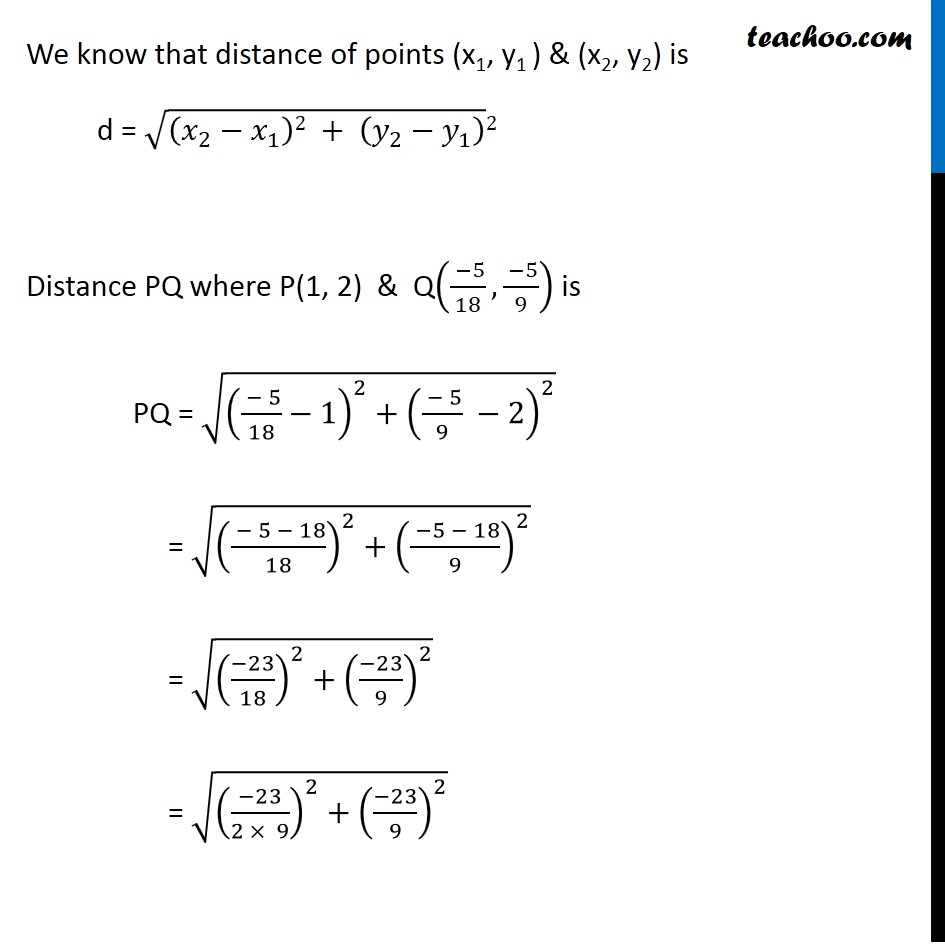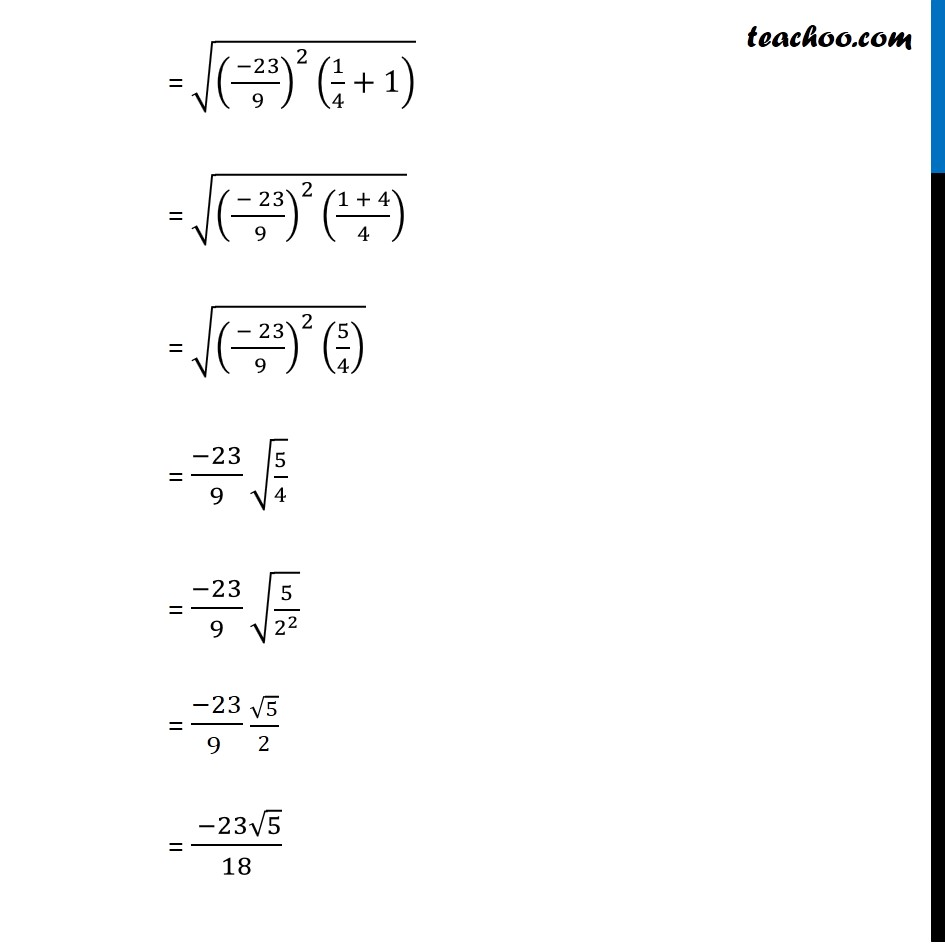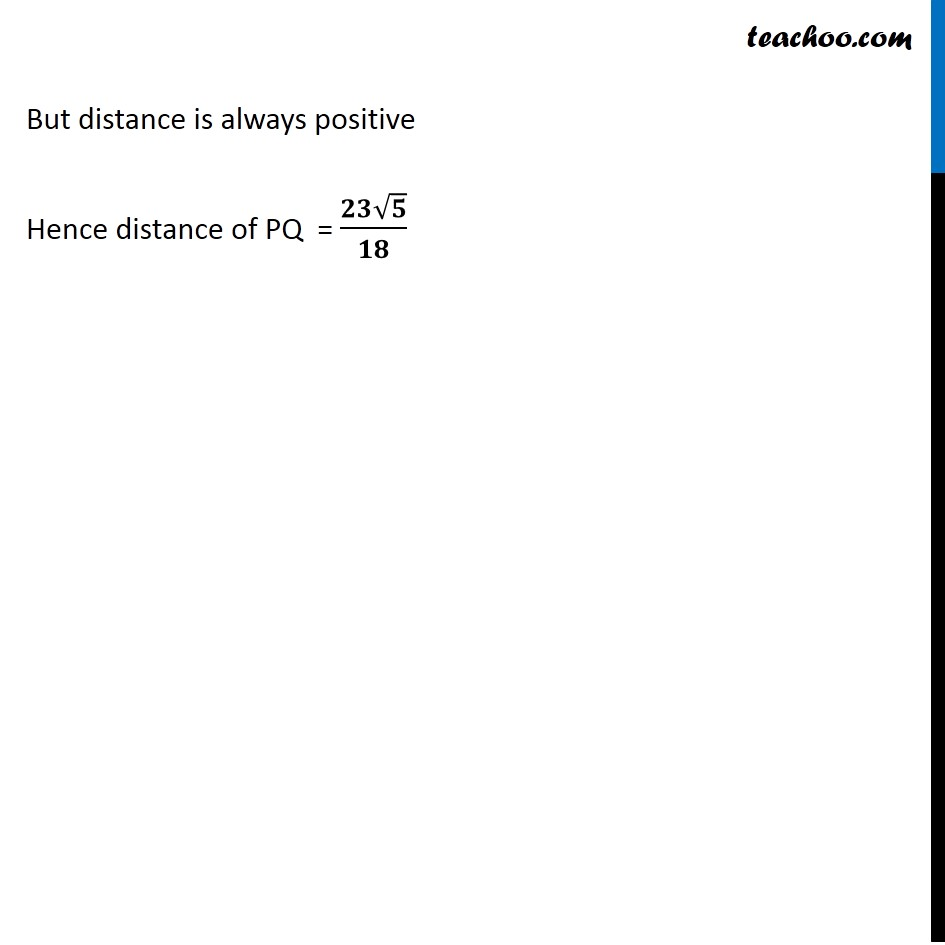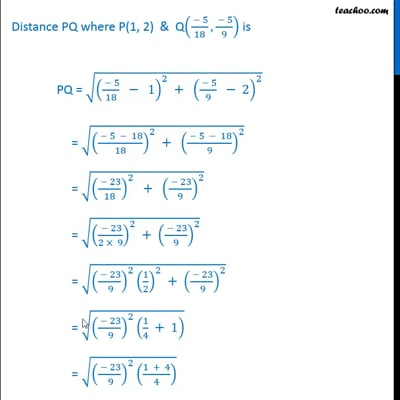This video is only available for Teachoo black users

Introducing your new favourite teacher - Teachoo Black, at only ₹83 per month

### Transcript

Misc 15 Find the distance of the line 4x + 7y + 5 = 0 from the point (1, 2) along the line 2x – y = 0. There are two lines Line AB 4x + 7y + 5 = 0 Line CD 2x – y = 0 Both lines meet at Q Point P(1, 2) is on line CD We need to find distance PQ. In PQ, P is (1, 2) We need to find point Q To find PQ, we must find coordinates of point Q Finding coordinate of point Q Point Q is the intersection of lines AB & CD From (1) 2x – y = 0 2x = y y = 2x Putting value of y in (2) 4x + 7(2x) + 5 = 0 4x + 14x + 5 = 0 18x = –5 x = (−5)/18 Putting x = (−5)/18 in (1) 2x – y = 0 2 ((−5)/18) – y = 0 (−5)/9 – y = 0 (−5)/9 = y y = (−5)/9 Hence coordinates of point Q is (( −𝟓)/𝟏𝟖,( −𝟓)/𝟗) Now, we have to find distance PQ We know that distance of points (x1, y1 ) & (x2, y2) is d = √((𝑥_2−𝑥_1 )2 + (𝑦_2−𝑦_1 ) ) 2 Distance PQ where P(1, 2) & Q(( −5)/18, ( −5)/9) is PQ = √((( − 5)/18−1)^2+(( − 5)/9 −2)^2 ) = √((( − 5 − 18)/18)^2+(( −5 − 18)/9)^2 ) = √(((−23)/18)^2+((−23)/9)^2 ) = √(((−23)/(2 × 9))^2+((−23)/9)^2 ) = √((( −23)/( 9))^2 (1/4+1) ) = √((( − 23)/( 9))^2 ((1 + 4)/4) ) = √((( − 23)/( 9))^2 (5/4) ) = (−23)/9 √(5/4) = (−23)/9 √(5/2^2 ) = (−23)/(9 ) √5/2 = ( −23√5)/18 But distance is always positive Hence distance of PQ = (𝟐𝟑√𝟓)/𝟏𝟖